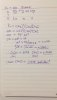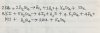# Chemistry 9701 Multiple choice questions

#### [email protected]

In which species is there a lone pair of electrons?
A. CH3
B. CH3+
C. CH3–
D. CH4

In CH3- carbon gets one extra electron as shown by negative charge and while it make 3 bonds one electron is left already so those both electrons make one lone pair in CH3-.

•nickrow66 and vedette

#### vedette

An excess of chlorine was bubbled into 100 cm3 of hot 6.0 mol dm–3 sodium hydroxide.
How many moles of sodium chloride would be produced in the reaction?
A 0.3
B 0.5
C 0.6
D 1.2

I need the working and explanation for this please.(the answer is B)
i finally found the solution.first off all write the balanced equation.it's going to give you:
6NaOH + 3Cl2 → 5NaCl + NaClO3 + 3H2O
then cal the no of mol in NaOH
n of moles = conc x vol= 0.6 x 100/1000=0.6
use the equation to find the no of mol in nacl
6→ 5
0.6→ x
=0.6 x 5/6=0.5 mol

#### Salome Ogunmodede

The reaction between sulfur dioxide and oxygen is reversible.
2SO2(g) + O2(g) 2SO3(g) Kc = 280 mol–1 dm3 at 1000K
In an equilibrium mixture at 1000K the sulfur trioxide concentration is 6.00mol dm–3.
The sulfur dioxide concentration is twice the oxygen concentration.
What is the sulfur dioxide concentration?
A 0.175 mol dm–3
B 0.254 mol dm–3
C 0.318 mol dm–3
D 0.636 mol dm–3

#### Salome Ogunmodede

The reaction between sulfur dioxide and oxygen is reversible.
2SO2(g) + O2(g) 2SO3(g) Kc = 280 mol–1 dm3 at 1000K
In an equilibrium mixture at 1000K the sulfur trioxide concentration is 6.00mol dm–3.
The sulfur dioxide concentration is twice the oxygen concentration.
What is the sulfur dioxide concentration?
A 0.175 mol dm–3
B 0.254 mol dm–3
C 0.318 mol dm–3
D 0.636 mol dm–3

I need the explanation and full method

#### nickrow66

The reaction between sulfur dioxide and oxygen is reversible.
2SO2(g) + O2(g) 2SO3(g) Kc = 280 mol–1 dm3 at 1000K
In an equilibrium mixture at 1000K the sulfur trioxide concentration is 6.00mol dm–3.
The sulfur dioxide concentration is twice the oxygen concentration.
What is the sulfur dioxide concentration?
A 0.175 mol dm–3
B 0.254 mol dm–3
C 0.318 mol dm–3
D 0.636 mol dm–3

The answer is D. Kc = [SO3]2/[SO2]2[O2], we are told the [SO2] is double that of [O2], so I wrote that as 2x and x respectively, not forgetting the power for [SO2], giving 4x2 and x. Therefore, Kc = 2/[4x3] giving us 280 = 36/4x3.

Therefore, x3 = 36/280 x 4 and take the cube root to find x, giving us the concentration of oxygen. Then double x for the concentration of SO2.

x = 0.3178 and 2x = 0.6356 or 0.636 mol/dm3

See attached.Last edited:

#### nickrow66

i think well you say the o2 conc is x and the so2 conc is 2x so i guess you substitute as follows and get your answer
kc= [s03]/x[s02]^2
A small quantity of hot, concentrated sulfuric acid is added separately to solid samples of potassium halides, KX.
Which potassium halides react and produce a mixture of products that include a halogen, X2?
1 potassium iodide
2 potassium bromide
3 potassium chloride

(the answer is saying 1 and 2 are correct). I need anexplanation for this please
The bromide ions are strong enough reducing agents to reduce the concentrated sulphuric acid. In the process, the bromide ions are oxidised to bromine. Iodide ions are stronger reducing agents than bromide ions are. They are oxidised to iodine by the concentrated sulphuric acid. Concentrated sulphuric acid is not a strong enough oxidising agent to oxidise fluoride or chloride ions.